NEET  >  Test: Units & Measurement - 3

# Test: Units & Measurement - 3

Test Description

## 25 Questions MCQ Test Physics Class 11 | Test: Units & Measurement - 3

Test: Units & Measurement - 3 for NEET 2022 is part of Physics Class 11 preparation. The Test: Units & Measurement - 3 questions and answers have been prepared according to the NEET exam syllabus.The Test: Units & Measurement - 3 MCQs are made for NEET 2022 Exam. Find important definitions, questions, notes, meanings, examples, exercises, MCQs and online tests for Test: Units & Measurement - 3 below.
Solutions of Test: Units & Measurement - 3 questions in English are available as part of our Physics Class 11 for NEET & Test: Units & Measurement - 3 solutions in Hindi for Physics Class 11 course. Download more important topics, notes, lectures and mock test series for NEET Exam by signing up for free. Attempt Test: Units & Measurement - 3 | 25 questions in 52 minutes | Mock test for NEET preparation | Free important questions MCQ to study Physics Class 11 for NEET Exam | Download free PDF with solutions
 1 Crore+ students have signed up on EduRev. Have you?
Test: Units & Measurement - 3 - Question 1

### Which of the following sets cannot enter into the list of fundamental quantities in any system of units ?

Detailed Solution for Test: Units & Measurement - 3 - Question 1

We define length and time separately as it is not possible to define velocity without using these quantities. This means that one fundamental quantity depends on the other. So, these quantities cannot be listed as fundamental quantities in any system of units.

Test: Units & Measurement - 3 - Question 2

### Which of the following is not the unit of time

Detailed Solution for Test: Units & Measurement - 3 - Question 2

Parallactic second is the unit of distance because parallactic second is an abbreviation of parsec. Parsec = Parsec is the Unit for larger distances.It is the distance at which a star would make parallax of one Second of arc.

Test: Units & Measurement - 3 - Question 3

### The unit of impulse is the same as that of :

Detailed Solution for Test: Units & Measurement - 3 - Question 3

The newton second (also newton-second, symbol N s or N.s) is the derived SI unit of impulse. It is dimensionally equivalent to the momentum unit kilogram metre per second (kg.m/s). One newton second corresponds to a one-newton force applied for one second.

Test: Units & Measurement - 3 - Question 4

Which of the following is not the unit of energy?

Detailed Solution for Test: Units & Measurement - 3 - Question 4

F = ma
[F] = kg m/s2

Test: Units & Measurement - 3 - Question 5

If the unit of length is micrometer and the unit of time is microsecond, the unit of velocity will be :

Detailed Solution for Test: Units & Measurement - 3 - Question 5

Unit of velocity = m/s
1m = 106  Micro meter
And 1 sec = 106  Micro sec
Thus 1 m/s = 106 106  micro meter /micro second

Test: Units & Measurement - 3 - Question 6

What is the physical quantity whose dimensions are M L2 T-2 ?

Detailed Solution for Test: Units & Measurement - 3 - Question 6

Dimension of KE is ML2T-2
That of pressure is ML-2T-2
That of momentum is ML/T
That of power is ML2T-3

Test: Units & Measurement - 3 - Question 7

A unitless quantity :

Detailed Solution for Test: Units & Measurement - 3 - Question 7

A dimensionless quantity can have unit. for example angle (radian). But oppisite is not true. A unitless quantity can never have dimensions.it is the unit that give dimensions.

Test: Units & Measurement - 3 - Question 8

If a and b are two physical quantities having different dimensions then which of the following can denote a new physical quantity

Detailed Solution for Test: Units & Measurement - 3 - Question 8

Quantities with different dimensions cant be added or subtracted. Also the dimension of the product of the quantities in power is always one. But when two quantities are multiplied the dimension of the product is the product of the dimensions of the initial quantities.

Test: Units & Measurement - 3 - Question 9

Two physical quantities whose dimensions are not same, cannot be :

Detailed Solution for Test: Units & Measurement - 3 - Question 9

The two physical quantities which do not have the same dimensions can’t be added or subtracted in the same expression because doing so would lead to equating two quantities of different dimensions, which is non possible. Ex [F]  [P].

Test: Units & Measurement - 3 - Question 10

Choose the correct statement (S)

Detailed Solution for Test: Units & Measurement - 3 - Question 10

Correct Answer :- d

Explanation : a) A topological sort of a directed acyclic graph (DAG) is any ordering m1, m2, …, mn of the nodes

of the graph, such that if mimj is an edge then mi appears before mj . Any topological sort of a

the dependency graph gives a valid evaluation order for the semantic rules.

b) The parse tree can be annotated with synthesized or inherited attributes. The parse tree can also be indicated with an arrow mark to indicate the manner in which the value gets propagated between the nodes of the parse tree. This graph is called as dependency graph as it indicates the dependency between nodes for deriving the values. This graph is an acyclic graph which doesn’t have a cycle. The presence of a cycle indicates that the graph is incorrect as the dependence of nodes for deriving values cannot be predicted. Edges in the dependence graph show the evaluation order for attribute values and thus the graph is a directed one.

Test: Units & Measurement - 3 - Question 11

Planck's constant has the dimensions of :

Detailed Solution for Test: Units & Measurement - 3 - Question 11

We know that E = hv , where E is energy and v is frequency.
Thus we get h = E/v
And [h] = [E/v] = ML2T-2 / T-1
= ML2T-1
[P] = MLT-1
[F] = MLT-2
[Angular momentum] = [P x r] = ML2T-1

Test: Units & Measurement - 3 - Question 12

Electron volt is a unit of

Test: Units & Measurement - 3 - Question 13

The dimensional formula of RT is same as that of:

Detailed Solution for Test: Units & Measurement - 3 - Question 13

The vander Waals gas equation is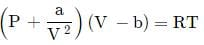where P is the pressure, V is molar volume and T is the temperature of the given sample of gas. R is called mola gas constant, a and b are called vander Waals constants.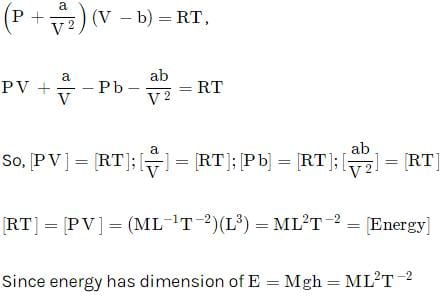Test: Units & Measurement - 3 - Question 14

Which pair of following quantities has dimensions different from each other

Detailed Solution for Test: Units & Measurement - 3 - Question 14

Moment of inertia =1/2​×mass×(Radius of gyration)2=[M1L2T0]
Moment of force = torque =N.m=[M1L2T−2]
Both are different from each other.

Test: Units & Measurement - 3 - Question 15

If the error in measurement of radius of sphere is 1% , what will be the error in measurement of volume

Detailed Solution for Test: Units & Measurement - 3 - Question 15

Volume=4πR3/3
ΔV/V×100=3∆R/R×100
=3×1/100​×100
=3
∴ Percentage error in volume is 3%

Test: Units & Measurement - 3 - Question 16

The velocity 'v' (in cm/s) of a particle is given in terms of time 't' (in s) by the equation

v = at +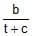The dimensions of a, b and c are

Detailed Solution for Test: Units & Measurement - 3 - Question 16

As v = at + b / (t + c)
We get that v, at and b / (t+c) have the same dimensions as they are equated and added.
Similarly c and t have also same dimensions, [c] = T and [a] = L/T
And [b] = L

Test: Units & Measurement - 3 - Question 17

In case of measurement of ‘g’, if error in measurement of length of pendulum is 2%, the percentage error in time period is1 %. The maximum error in measurement of g is

Detailed Solution for Test: Units & Measurement - 3 - Question 17

Time period of oscillation of pendulam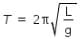where L is length of pendulam and g is acceleration due to gravity.
hence we have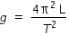...........................(1)
hence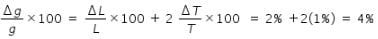Test: Units & Measurement - 3 - Question 18

The time dependence of a physical quantity ?

P = P0exp(_at2)

where a is a constant and t is time. The constant a

Detailed Solution for Test: Units & Measurement - 3 - Question 18

We know that any quantity in power as a dimension of 1, thus [at2] = 1
Thus we get [a] = T-2

Test: Units & Measurement - 3 - Question 19

Force F is given in terms of time t and distance x by

F = A sin C t + B cos D x

Then the dimensions of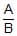and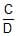are given by

Detailed Solution for Test: Units & Measurement - 3 - Question 19

Dimension of [A]=[MLT-2]
Dimension of [B]=[MLT-2]

[A]/[B]= [ M0L0T0]
CT=1
Dimension of  [C]=1/[T]=[T-1]
DL=1
Dimension of  [D]=1/[L]=[L-1]
[C][D] =[T-1]/[L-1]
=LT-1

A/B=Force/Force=[M0L0T0]
Ct=∠⇒C=Angle/Time=1/T=T-1
Dx=∠⇒D=Angle/Distance=1/L=L-1
∴C/D=T-1L-1 =[M0 LT-1 ]

Test: Units & Measurement - 3 - Question 20

The Van der Waal equation for 1 mole of a real gas is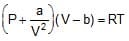where P is the pressure, V is the volume, T is the absolute temperature, R is the molar gas constant and a, b are Van dar Waal constants. The dimensions of a are the same as those of

Detailed Solution for Test: Units & Measurement - 3 - Question 20

The answer is PV2
Solution,
As we know the Vander Waals equation is {P+(a/V2)} (V−b) =RT
Then,
The dimension of a is,
(a/V2) = (P)
Or, a=PV2

Test: Units & Measurement - 3 - Question 21

The dimensional formula of coefficient of viscosity is

Test: Units & Measurement - 3 - Question 22

If force (F) is given by F = Pt_1 + Qt, where t is time. The unit of P is same as that of

Detailed Solution for Test: Units & Measurement - 3 - Question 22

According to the principle of homogeneity of dimension, an equation is dimensionally correct when each term has same dimension on both sides of the equation.

Since left side has dimension of force so the term Pt−1 will have also dimension of force.

Thus, [P][T−1]=[F]=[MLT−2]

or [P]=[MLT−1]

We know that the dimension of momentum is [p]=[MLT−1]

Test: Units & Measurement - 3 - Question 23

The product of energy and time is called action. The dimensional formula for action is same as that for

Detailed Solution for Test: Units & Measurement - 3 - Question 23

Both are different from each other.
Energy × Time=(M1L2T−2)×(T1)=M1L2T−1
Force × Velocity=(M1L1T−2)×(L1T−1)=M1L2T−3
Impulse × distance=(M1L1T−1)×(L1)=M1L2T−1
Power=M1L2T−3
Angular Energy=M1L1T−2

Test: Units & Measurement - 3 - Question 24

When a wave traverses a medium, the displacement of a particle located at x at time t is given by y = a sin (bt - cx) where a, b and c are constants of the wave. The dimensions of b/c are the same as those of

Detailed Solution for Test: Units & Measurement - 3 - Question 24

Argument of sin is dimensionless and using principle of dimensional homogeneity, we get
∣bt∣=∣cx∣=[M0L0T0]
Thus, ∣b∣=T−1 and ∣c∣=[L−1]
Thus, dimensions of b/c are [LT−1], which is the same as the velocity of wave.

Test: Units & Measurement - 3 - Question 25

In the above question dimensions of b/c are the same as those of

## Physics Class 11

126 videos|449 docs|210 tests
 Use Code STAYHOME200 and get INR 200 additional OFF Use Coupon Code
Information about Test: Units & Measurement - 3 Page
In this test you can find the Exam questions for Test: Units & Measurement - 3 solved & explained in the simplest way possible. Besides giving Questions and answers for Test: Units & Measurement - 3, EduRev gives you an ample number of Online tests for practice

## Physics Class 11

126 videos|449 docs|210 tests

### How to Prepare for NEET

Read our guide to prepare for NEET which is created by Toppers & the best Teachers